Students can download Maths Chapter 5 Coordinate Geometry Ex 5.1 Questions and Answers, Notes, Samacheer Kalvi 10th Maths Guide Pdf helps you to revise the complete Tamilnadu State Board New Syllabus, helps students complete homework assignments and to score high marks in board exams.

## Tamilnadu Samacheer Kalvi 10th Maths Solutions Chapter 5 Coordinate Geometry Ex 5.1

Question 1.
Find the area of the triangle formed by the points
(i) (1,-1), (-4, 6) and (-3, -5)
(ii) (-10, -4), (-8, -1) and (-3, -5)
Solution:
(i) Let the vertices A (1, -1), B (-4, 6) and C (-3, -5)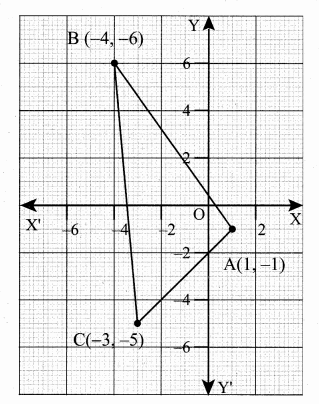= $$\frac { 1 }{ 2 }$$ [(6 + 20 + 3) – (4 – 18 – 5)] = $$\frac { 1 }{ 2 }$$ [29 – (-19)] = $$\frac { 1 }{ 2 }$$ [29 + 19]
= $$\frac { 1 }{ 2 }$$ × 48 = 24 sq. units.
Area of ∆ABC = 24 sq. units(ii) Let the vertices be A(-10, -4), B(-8 -1) and C(-3, -5)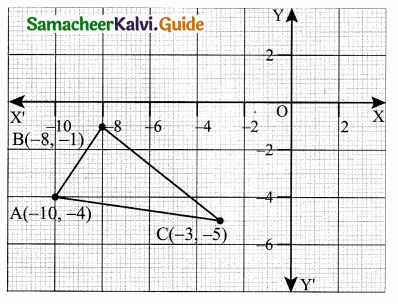Area of ∆ABC = $$\frac { 1 }{ 2 }$$[(x1y2 + x2y3 + x3y1) – (x2y1 + x3y2 + x1y3)]
= $$\frac { 1 }{ 2 }$$ [(50 + 3 + 32) – (12 + 40 + 10)]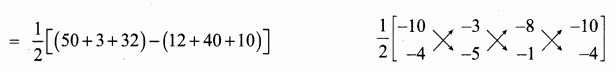= $$\frac { 1 }{ 2 }$$ [85 – (62)] = $$\frac { 1 }{ 2 }$$  = 11.5
Area of ∆ACB = 11.5 sq.unitsQuestion 2.
Determine whether the sets of points are collinear?
(i) (-$$\frac { 1 }{ 2 }$$,3)
(ii) (a,b + c), (b,c + a) and (c,a + b)
Solution:
(i) Let the points be A (-$$\frac { 1 }{ 2 }$$,3), B (-5, 6) and C(-8, 8)
Area of ∆ABC = $$\frac { 1 }{ 2 }$$ [(x1y2 + x2y3 + x3y1) – (x2y1 + x3y3 + x1y3)]
= $$\frac { 1 }{ 2 }$$ [(- 3 – 40 – 24) – (-15 – 48 – 4)]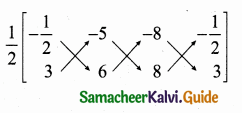= $$\frac { 1 }{ 2 }$$ [-67 + 67] = $$\frac { 1 }{ 2 }$$ × 0 = 0
Area of a ∆ is 0.
∴ The three points are collinear.

(ii) Let the points be A (a, b + c), B (b, c + a) and C (c, a + b)
Area of the triangle = $$\frac { 1 }{ 2 }$$ [(x1y2 + x2y3 + x3y1) – (x2y1 + x3y2 + x1y3)]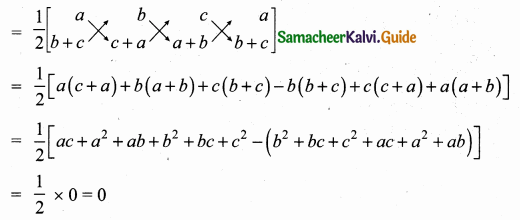Since the area of a triangle is 0.
∴ The given points are collinear.Question 3.
Vertices of given triangles are taken in order and their areas are provided aside. In each case, find the value of ‘p’Solution:
(i) Let the vertices be A (0,0) B (p, 8), c (6, 2)
Area of a triangle = 20 sq. units
$$\frac { 1 }{ 2 }$$ [(x1y2 + x2y3 + x3y1) – (x2y1 + x3y2 + x1y3)] = 20$$\frac { 1 }{ 2 }$$ [(0 + 2p + 0) – (0 + 48 + 0)] = 20
$$\frac { 1 }{ 2 }$$ [2p – 48] = 20
2p – 48 = 40 ⇒ 2p = 40 + 48
p = $$\frac { 88 }{ 2 }$$ = 44
The value of p = 44

(ii) Let the vertices be A (p, p), B (5, 6) and C (5, -2)
Area of a triangle = 32 sq. units
$$\frac { 1 }{ 2 }$$ [(x1y2 + x2y3 + x3y1) – (x2y1 + x3y2 + x1y3)] = 32$$\frac { 1 }{ 2 }$$ [6p – 10 + 5p) – (5p + 30 – 2p)] = 32
$$\frac { 1 }{ 2 }$$ [11 p – 10 – 3p – 30] = 32
11p – 10 – 3p – 30 = 64
8p – 40 = 64
8p = 64 + 40 ⇒ 8p = 104
p = $$\frac { 104 }{ 8 }$$ = 13
The value of p = 13Question 4.
In each of the following, find the value of ‘a’ for which the given points are collinear.
(i) (2,3), (4, a) and (6, -3)
(ii) (a, 2 – 2a), (-a + 1, 2a) and (-4 -a, 6 – 2a).
Solution:
(i) Let the points be A (2, 3), B(4, a) and C(6, -3).
Since the given points are collinear.
Area of a triangle = 0
$$\frac { 1 }{ 2 }$$ [(x1y2 + x2y3 + x3y1) – (x2y1 + x3y2 + x1y3)] = 0)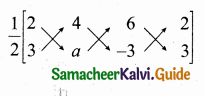$$\frac { 1 }{ 2 }$$ [(2a – 12 + 18) – (12 + 6a – 6)] = 0
2a + 6 – (6 + 6a) = 0
2a + 6 – 6 – 6a = 0
-4a = 0 ⇒ a = $$\frac { 0 }{ 4 }$$ = 0
The value of a = 0

(ii) Let the points be A (a, 2 – 2a), B (-a + 1, 2a) C (-4 -a, 6 – 2a).
Since the given points are collinear.
Area of a ∆ = 0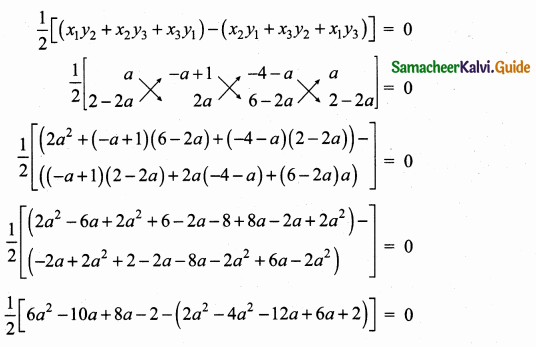6a2 – 2a – 2 – (-2a2 – 6a + 2) = 0
6a2 – 2a – 2 + 2a2 + 6a – 2 = 0
8a2 + 4a – 4 = 0 (Divided by 4)
2a2 + a – 1 = 0
2a2 + 2a – a – 1 = 0
2a (a + 1) – 1 (a + 1) = 0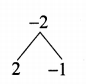(a + 1) (2a – 1) = 0
a + 1 = 0 (or) 2a – 1 = 0
a = -1 (or) 2a = 1 ⇒ a = $$\frac { 1 }{ 2 }$$
The value of a = -1 (or) $$\frac { 1 }{ 2 }$$Question 5.
Find the area of the quadrilateral whose vertices are at
(i) (-9, -2), (-8, -4), (2, 2) and (1, -3)
(ii) (-9, 0), (-8,6), (-1, -2) and (-6, -3)
Solution:
(i) Let the vertices A (-9, -2), B(-8, -4), C(2, 2) and D(1, -3).
Plot the vertices in a graph.[Note: Consider the points in counter clock wise order]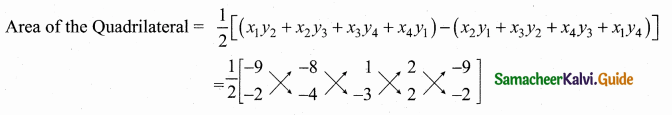Area of the Quadrilateral ABDC = $$\frac { 1 }{ 2 }$$ [36 + 24 + 2 – 4 – (16 – 4 – 6 – 18)]
= $$\frac { 1 }{ 2 }$$ [58 – (-12)] – $$\frac { 1 }{ 2 }$$[58 + 12]
= $$\frac { 1 }{ 2 }$$ × 70 = 35 sq. units 2
Area of the Quadrilateral = 35 sq. units(ii) Let the vertices A(-9, 0), B(-8, 6), C(-1, -2) and D(-6, -3)
Plot the vertices in a graph and take them in counter – clock wise order.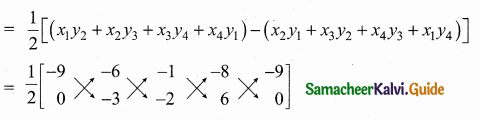= $$\frac { 1 }{ 2 }$$ [33 + 35] = $$\frac { 1 }{ 2 }$$ × 68 = 34 sq. units
Area of the Quadrilateral = 34 sq. unitsQuestion 6.
Find the value of k, if the area of a quadrilateral is 28 sq. units, whose vertices are (-4, -2), (-3, k), (3, -2) and (2, 3)
Solution:
Let the vertices A (-A, -2), B (-3, k), C (3, -2) and D (2, 3)
Area of the Quadrilateral = 28 sq. units
$$\frac { 1 }{ 2 }$$ [(x1y2 + x2y3 + x3y4 + x4y1) – (x2y1 + x3y2 + x4y3 + x1y4)] = 28-7k + 21 = 56
-7k = 56 – 21
-7k = 35 ⇒ 7k = – 35
k = – $$\frac { 35 }{ 7 }$$ = -5
The value of k = -5Question 7.
If the points A(-3, 9), B(a, b) and C(4, -5) are collinear and if a + b = 1, then find a and b.
Solution:
Since the three points are collinear
Area of a ∆ = 0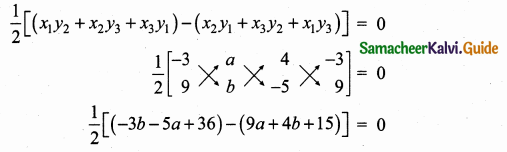-3b – 5a + 36 – 9a – 4b – 15 = 0
-7b – 14a + 21 = 0
(÷ by 7) – b – 2a + 3 = 0
2a + b – 3 = 0Substitute the value of a = 2 in (2) ⇒ 2 + b = 1
b = 1 – 2 = -1
The value of a = 2 and b = -1Question 8.
Let P(11, 7), Q(13.5, 4) and R(9.5, 4) be the midpoints of the sides AB, BC and AC respectively of ∆ABC . Find the coordinates of the vertices A, B and C. Hence find the area of ∆ABC and compare this with area of ∆PQR.
Solution:
Let the vertices of the ∆ABC be A(x1,y1), B(x2,y2), C(x3,y3)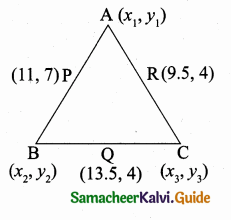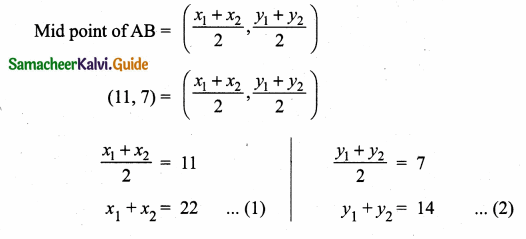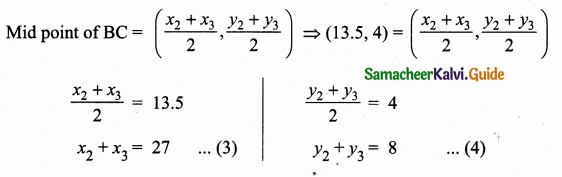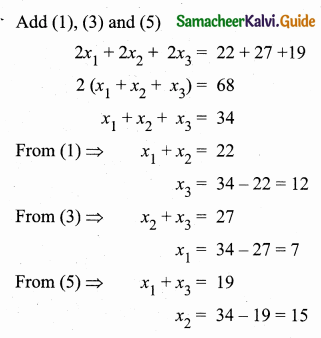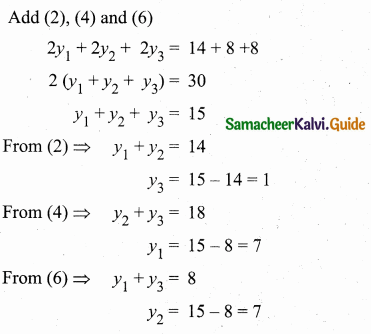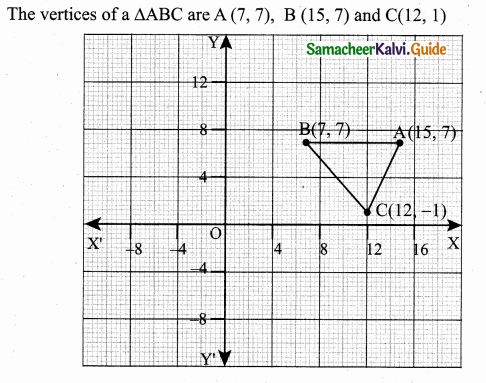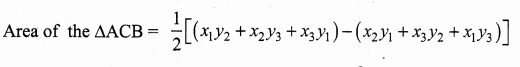Question 9.
In the figure, the quadrilateral swimming pool shown is surrounded by concrete patio. Find the area of the patio.Solution: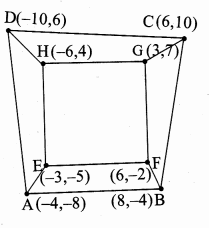= $$\frac { 1 }{ 2 }$$ [(x1y2 + x2y3 + x3y4 + x4y1) – (x2y1 + x3y2 + x4y3 + x1y4)]
= $$\frac { 1 }{ 2 }$$ [(16 + 80 + 36 + 80) – (-64 – 24 – 100 – 24)]
= $$\frac { 1 }{ 2 }$$ [212 – (-212)]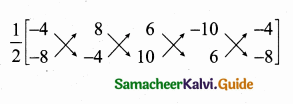= $$\frac { 1 }{ 2 }$$ [212 + 212] = $$\frac { 1 }{ 2 }$$  = 212 sq. units= $$\frac { 1 }{ 2 }$$ [90 – (-90)]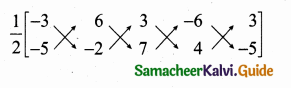= $$\frac { 1 }{ 2 }$$ [90 + 90]
= $$\frac { 1 }{ 2 }$$ × 180 = 90 sq. units
Area of the patio = Area of the Quadrilateral ABCD – Area of the Quadrilateral EFGH
= (212 – 90) sq. units
Area of the patio = 122 sq. unitsQuestion 10.
A triangular shaped glass with vertices at A(-5, -4), B(l, 6) and C(7, -4) has to be painted. If one bucket of paint covers 6 square feet, how many buckets of paint will be required to paint the whole glass, if only one coat of paint is applied.
Solution:
Given the vertices of the triangular glass is A (-5, -4), B (1, 6), and C (7, -4)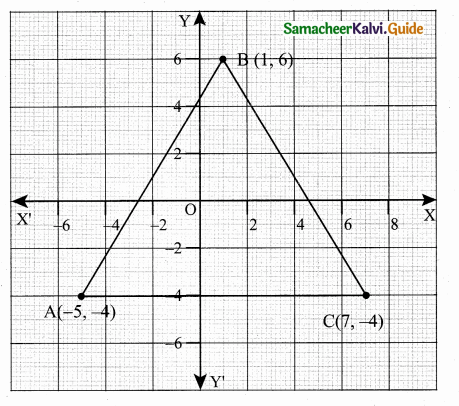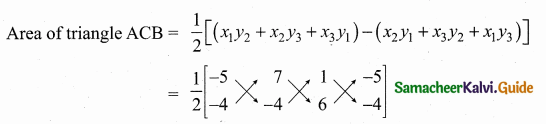= $$\frac { 1 }{ 2 }$$ [(20 + 42 – 4) – (-28 – 4 – 30)]
= $$\frac { 1 }{ 2 }$$ [58 – (-62)]
= $$\frac { 1 }{ 2 }$$ [58 + 62]
= $$\frac { 1 }{ 2 }$$ × 120 = 60 sq. feet
Number of cans to paint 6 square feet = 1
∴ Number of cans = $$\frac { 60 }{ 6 }$$ = 10 ⇒ Number of cans = 10Question 11.
In the figure, find the area of
(i) triangle AGF
(ii) triangle FED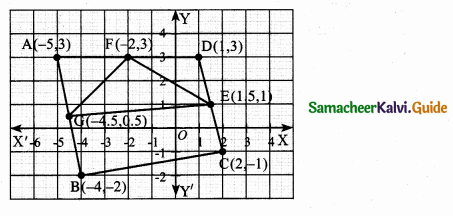Solution:
Area of a triangle = $$\frac { 1 }{ 2 }$$ [(x1y2 + x2y3 + x3y1) – (x2y1 + x3y2 + x1y3)]
(i) Area of ∆AGF = $$\frac { 1 }{ 2 }$$ [(-2.5 – 13.5 – 6) – (-13.5 – 1 – 15)]
= $$\frac { 1 }{ 2 }$$ [-22 – (-29.5)]= $$\frac { 1 }{ 2 }$$ [-22 + 29.5]
= $$\frac { 1 }{ 2 }$$ × 7.5 = 3.75 sq.units

(ii) Area of ∆FED = $$\frac { 1 }{ 2 }$$ [(-2 + 4.5 + 3) – (4.5 + 1 – 6)]
= $$\frac { 1 }{ 2 }$$ [5.5 – (-0.5)]= $$\frac { 1 }{ 2 }$$ [5.5 + 0.5] = $$\frac { 1 }{ 2 }$$ × 6 = 3 sq.units

(iii)= $$\frac { 1 }{ 2 }$$ [(4 + 2 + 0.75 + 9) – (-4 -1.5 – 4.5 -2)]
= $$\frac { 1 }{ 2 }$$ [15.75 + 12]
= $$\frac { 1 }{ 2 }$$ [27.75] = 13.875
= 13.88 sq. units